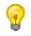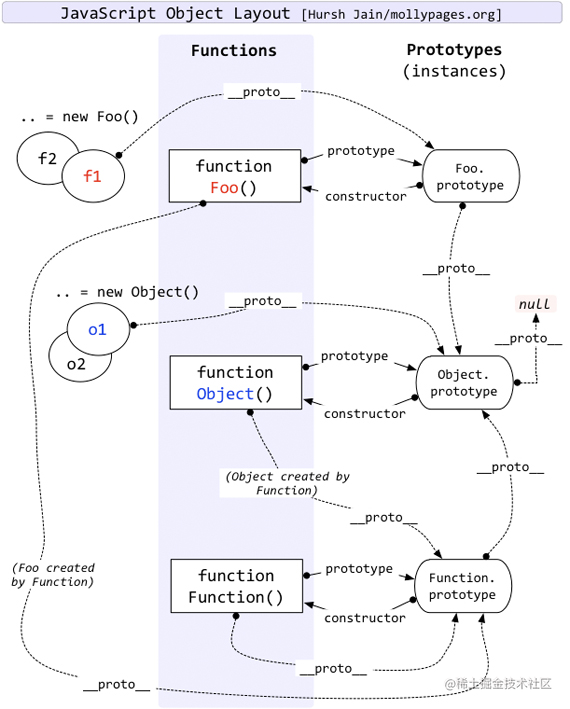# JavaScript 类型转换与检测

JavaScript是一门弱类型（或称动态类型）的语言，即变量的类型是不确定的。下面通过本文给大家分享javascript数据类型转换小结，包括显式数据类型转换和隐式数据类型转换

### typeof 操作符

``````typeof myCar                  // 返回 undefined (如果 myCar 没有声明)
typeof "John"                 // 返回 string
typeof 3.14                   // 返回 number
typeof NaN                    // 返回 number
typeof false                  // 返回 boolean
typeof null                   // 返回 object
typeof [1,2,3,4]              // 返回 object
typeof {name:'John', age:34}  // 返回 object
typeof new Date()             // 返回 object
typeof function () {}         // 返回 function注意: 如果对象是 JavaScript Array 或 JavaScript Date ，我们就无法通过 typeof 来判断他们的类型，因为都是返回object

• 未定义变量的数据类型为 undefined
• NaN 的数据类型是 number
• 数组(Array)的数据类型是 object
• 日期(Date)的数据类型为 object
• null 的数据类型是 object

### constructor 属性

constructor 属性返回所有 JavaScript 变量的构造函数

``````"John".constructor                 // 返回函数 String()  { [native code] }
(3.14).constructor                 // 返回函数 Number()  { [native code] }
false.constructor                  // 返回函数 Boolean() { [native code] }

function () {}.constructor         // 返回函数 Function(){ [native code] }
[1,2,3,4].constructor              // 返回函数 Array()   { [native code] }
{name:'John', age:34}.constructor  // 返回函数 Object()  { [native code] }
new Date().constructor             // 返回函数 Date()    { [native code] }

``````function isType(type) {
return function (obj) {
return obj.constructor.toString().indexOf(type) > -1;
}
}

//你可以使用 constructor 属性来查看对象是否为数组 (包含字符串 "Array"):
var isArray = isType("Array")
console.log(isArray([1, 2, 3])) //true

//你可以使用 constructor 属性来查看对象是否为日期 (包含字符串 "Date"):
var isDate = isType("Date")
console.log(isDate(new Date())) //true

### instanceof 运算符深入剖析

``````var oStringObject = new String("hello world")
console.log(oStringObject instanceof String)      // 输出 "true"

#### instanceof 常规用法

``````// 判断 foo 是否是 Foo 类的实例
function Foo(){}
var foo = new Foo();
console.log(foo instanceof Foo)//true

#### instanceof 在继承中关系中的用法

``````// 判断 foo 是否是 Foo 类的实例 , 并且是否是其父类型的实例
function Aoo(){}
function Foo(){}
Foo.prototype = new Aoo();//JavaScript 原型继承

var foo = new Foo();
console.log(foo instanceof Foo)//true
console.log(foo instanceof Aoo)//true

#### instanceof 复杂用法

``````console.log(Object instanceof Object)       //true
console.log(Function instanceof Function)   //true

console.log(Number instanceof Number)       //false
console.log(String instanceof String)       //false

console.log(Function instanceof Object)     //true

function Foo() { }
console.log(Foo instanceof Function)        //true
console.log(Foo instanceof Foo)             //false

• 语言规范中是如何定义这个运算符的
• JavaScript 原型继承机制

#### JavaScript instanceof 运算符代码

``````function instance_of(L, R) {//L 表示左表达式，R 表示右表达式
var O = R.prototype;// 取 R 的显示原型
L = L.__proto__;// 取 L 的隐式原型
while (true) {
if (L === null)
return false;
if (O === L)// 这里重点：当 O 严格等于 L 时，返回 true
return true;
L = L.__proto__;
}
}

#### JavaScript 原型继承机制#### Object instanceof Object

``````// 为了方便表述，首先区分左侧表达式和右侧表达式
ObjectL = Object, ObjectR = Object;
// 下面根据规范逐步推演
O = ObjectR.prototype = Object.prototype
L = ObjectL.__proto__ = Function.prototype
// 第一次判断
O != L
// 循环查找 L 是否还有 __proto__
L = Function.prototype.__proto__ = Object.prototype
// 第二次判断
O == L
// 返回 true

#### Function instanceof Function

``````// 为了方便表述，首先区分左侧表达式和右侧表达式
FunctionL = Function, FunctionR = Function;
// 下面根据规范逐步推演
O = FunctionR.prototype = Function.prototype
L = FunctionL.__proto__ = Function.prototype
// 第一次判断
O == L
// 返回 true

#### Foo instanceof Foo

``````// 为了方便表述，首先区分左侧表达式和右侧表达式
FooL = Foo, FooR = Foo;
// 下面根据规范逐步推演
O = FooR.prototype = Foo.prototype
L = FooL.__proto__ = Function.prototype
// 第一次判断
O != L
// 循环再次查找 L 是否还有 __proto__
L = Function.prototype.__proto__ = Object.prototype
// 第二次判断
O != L
// 再次循环查找 L 是否还有 __proto__
L = Object.prototype.__proto__ = null
// 第三次判断
L == null

#### Dojo 中多重继承

``````dojo.declare("Aoo",null,{});
dojo.declare("Boo",null,{});
dojo.declare("Foo",[Aoo,Boo],{});

var foo = new Foo();
console.log(foo instanceof Aoo);//true
console.log(foo instanceof Boo);//false

console.log(foo.isInstanceOf(Aoo));//true
console.log(foo.isInstanceOf(Boo));//true

### Object.prototype.toString

toString() 是 Object 的原型方法，调用该方法，默认返回当前对象的 [[Class]] 。这是一个内部属性，其格式为 [object Xxx] ，其中 Xxx 就是对象的类型 对于 Object 对象，直接调用 toString()  就能返回 [object Object] 。而对于其他对象，则需要通过 call apply来调用才能返回正确的类型信息

``````Object.prototype.toString.call('')             // [object String]
Object.prototype.toString.call(1)              // [object Number]
Object.prototype.toString.call(true)           // [object Boolean]
Object.prototype.toString.call(Symbol())       // [object Symbol]
Object.prototype.toString.call(undefined)      // [object Undefined]
Object.prototype.toString.call(null)           // [object Null]
Object.prototype.toString.call(new Function()) // [object Function]
Object.prototype.toString.call(new Date())     // [object Date]
Object.prototype.toString.call([])             // [object Array]
Object.prototype.toString.call(new RegExp())   // [object RegExp]
Object.prototype.toString.call(new Error())    // [object Error]
Object.prototype.toString.call(window)         // [object global] window 是全局对象 global 的引用
Object.prototype.toString.call(document)       // [object HTMLDocument]

``````function isType(type) {
return function(data) {
return Object.prototype.toString.call(data) == type //true
}
}

var isArray = isType("[object Array]")
var isDate = isType("[object Date]")

console.log(isArray([1,2,3]));
console.log(isDate(new Date()));

### JavaScript 类型转换

JavaScript 变量可以转换为新变量或其他数据类型：

• 通过使用 JavaScript 函数
• 通过 JavaScript 自身自动转换

#### 将其他类型转换为字符串

``````var x = 10
String(x)         // 将变量 x 转换为字符串并返回
String(123)       // 将数字 123 转换为字符串并返回
String(100 + 23)  // 将数字表达式转换为字符串并返回

Number 方法toString()也是有同样的效果

``````x.toString()
(123).toString()
(100 + 23).toString()

toExponential()把对象的值转换为指数计数法
toFixed()把 Number 四舍五入为指定小数位数的字符串把数字转换为字符串，结果的小数点后有指定位数的数字
toPrecision()把数字格式化为指定的长度

``````String(false)        // 返回 "false"
String(true)         // 返回 "true"

Boolean 方法toString()也有相同的效果

``````false.toString()     // 返回 "false"
true.toString()      // 返回 "true"

``````Date()                  // 返回 Thu Jul 17 2014 15:38:19 GMT+0200 (W. Europe Daylight Time)
String(new Date())      // 返回 Thu Jul 17 2014 15:38:19 GMT+0200 (W. Europe Daylight Time)

Date 方法toString()也有相同的效果

``````obj = new Date()
obj.toString()   // 返回 Thu Jul 17 2014 15:38:19 GMT+0200 (W. Europe Daylight Time)

Date 方法 章节中，你可以查看更多关于日期转换为字符串的函数：

getDate()从 Date 对象返回一个月中的某一天 (1 ~ 31)
getDay()从 Date 对象返回一周中的某一天 (0 ~ 6)
getFullYear()从 Date 对象以四位数字返回年份
getHours()返回 Date 对象的小时 (0 ~ 23)
getMilliseconds()返回 Date 对象的毫秒(0 ~ 999)
getMinutes()返回 Date 对象的分钟 (0 ~ 59)
getMonth()从 Date 对象返回月份 (0 ~ 11)
getSeconds()返回 Date 对象的秒数 (0 ~ 59)
getTime()返回 1970 年 1 月 1 日至今的毫秒数

** 一元运算符 Operator +可用于将变量转换为数字：

``````var y = "5";      // y 是一个字符串
var x = + y;      // x 是一个数字

``````var y = "John";   // y 是一个字符串
var x = + y;      // x 是一个数字 (NaN)

#### 将其他类型转换为数字

``````Number("3.14")    // 返回 3.14
Number(" ")       // 返回 0
Number("")        // 返回 0
Number("99 88")   // 返回 NaN

Number 方法 章节中，你可以查看到更多关于字符串转为数字的方法：

parseFloat()解析一个字符串，并返回一个浮点数
parseInt()解析一个字符串，并返回一个整数

js四舍五入保留两位小数，且不足补0

``````function fomatFloat(n, pos) {
var num = parseFloat(n)
if (isNaN(num)) return false

num = Math.round(num * Math.pow(10, pos)) / Math.pow(10, pos) // pow 幂
// num = Math.round(num * 10 ** pos) / 10 ** pos

var str = num.toString()
var str_l = str.indexOf('.')

if (str_l < 0 && pos > 0) {
str_l = str.length
str += '.'
}

while (str.length <= str_l + pos) { str += '0' }
return str
}
console.log("3.10159267保留2位小数：" + fomatFloat(3000.5005, 10))

``````Number(false)     // 返回 0
Number(true)      // 返回 1
Number("26.3aaa") // 结果NaN

``````d = new Date();
Number(d)          // 返回 1404568027739

``````d = new Date();
d.getTime()        // 返回 1404568027739

#### 将其他类型转换布尔值

``````Boolean(1)            //true
Boolean(11)           //true
Boolean(-10)          //true
Boolean("哈哈")        //true
Boolean("000")        //true
Boolean("0");         //true
Boolean("")           //flase
Boolean(-0)           //flase
Boolean(0)            //flase
Boolean(null)         //flase
Boolean(undefined)    //flase

#### 自动转换类型

``````5 + null    // 返回 5         null 转换为 0
"5" + null  // 返回"5null"    null 转换为 "null"
"5" + 1     // 返回 "51"      1 转换为 "1"
"5" - 1     // 返回 4         "5" 转换为 5

``````document.getElementById("demo").innerHTML = myVar;

myVar = {name:"Fjohn"}  // toString 转换为 "[object Object]"
myVar = [1,2,3,4]       // toString 转换为 "1,2,3,4"
myVar = new Date()      // toString 转换为 "Fri Jul 18 2014 09:08:55 GMT+0200"

``````myVar = 123             // toString 转换为 "123"
myVar = true            // toString 转换为 "true"
myVar = false           // toString 转换为 "false"

false0"false"false尝试一下 »
true1"true"true尝试一下 »
00"0"false尝试一下 »
11"1"true尝试一下 »
"0"0"0"true尝试一下 »
"000"0"000"true尝试一下 »
"1"1"1"true尝试一下 »
NaNNaN"NaN"false尝试一下 »
InfinityInfinity"Infinity"true尝试一下 »
-Infinity-Infinity"-Infinity"true尝试一下 »
""0""false尝试一下 »
"20"20"20"true尝试一下 »
"Runoob"NaN"Runoob"true尝试一下 »
[ ]0""true尝试一下 »
20"20"true尝试一下 »
[10,20]NaN"10,20"true尝试一下 »
["Runoob"]NaN"Runoob"true尝试一下 »